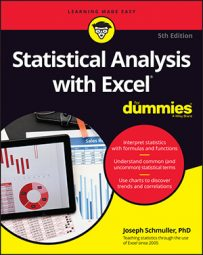##### Statistical Analysis with Excel For DummiesLinear regression is a great tool for making predictions with Excel. When you know the slope and the intercept of the line that relates two variables, you can take a new x-value and predict a new y-value. In the example you’ve been working through, you take a SAT score and predict a GPA for a Sahutsket University student.

What if you knew more than just the SAT score for each student? What if you had the student’s high-school average (on a 100 scale), and you could use that information, too? If you could combine SAT score with HS average, you might have a more accurate predictor than SAT score alone.

When you work with more than one independent variable, you’re in the realm of multiple regression. As in linear regression, you find regression coefficients for the best-fitting line through a scatterplot. Once again, best-fitting means that the sum of the squared distances from the data points to the line is a minimum.

With two independent variables, however, you can’t show a scatterplot in two dimensions. You need three dimensions, and that becomes difficult to draw.

For the SAT-GPA example, the regression equation translates to

Predicted GPA =a+b1(SAT)+b2(High School Average)

You can test hypotheses about the overall fit, and about all three of the regression coefficients.

Let’s check out the Excel capabilities for finding coefficients.

A few things to bear in mind:

• You can have any number of x-variables.
• Expect the coefficient for SAT to change from linear regression to multiple regression. Expect the intercept to change, too.
• Expect the standard error of estimate to decrease from linear regression to multiple regression. Because multiple regression uses more information than linear regression, it reduces the error.